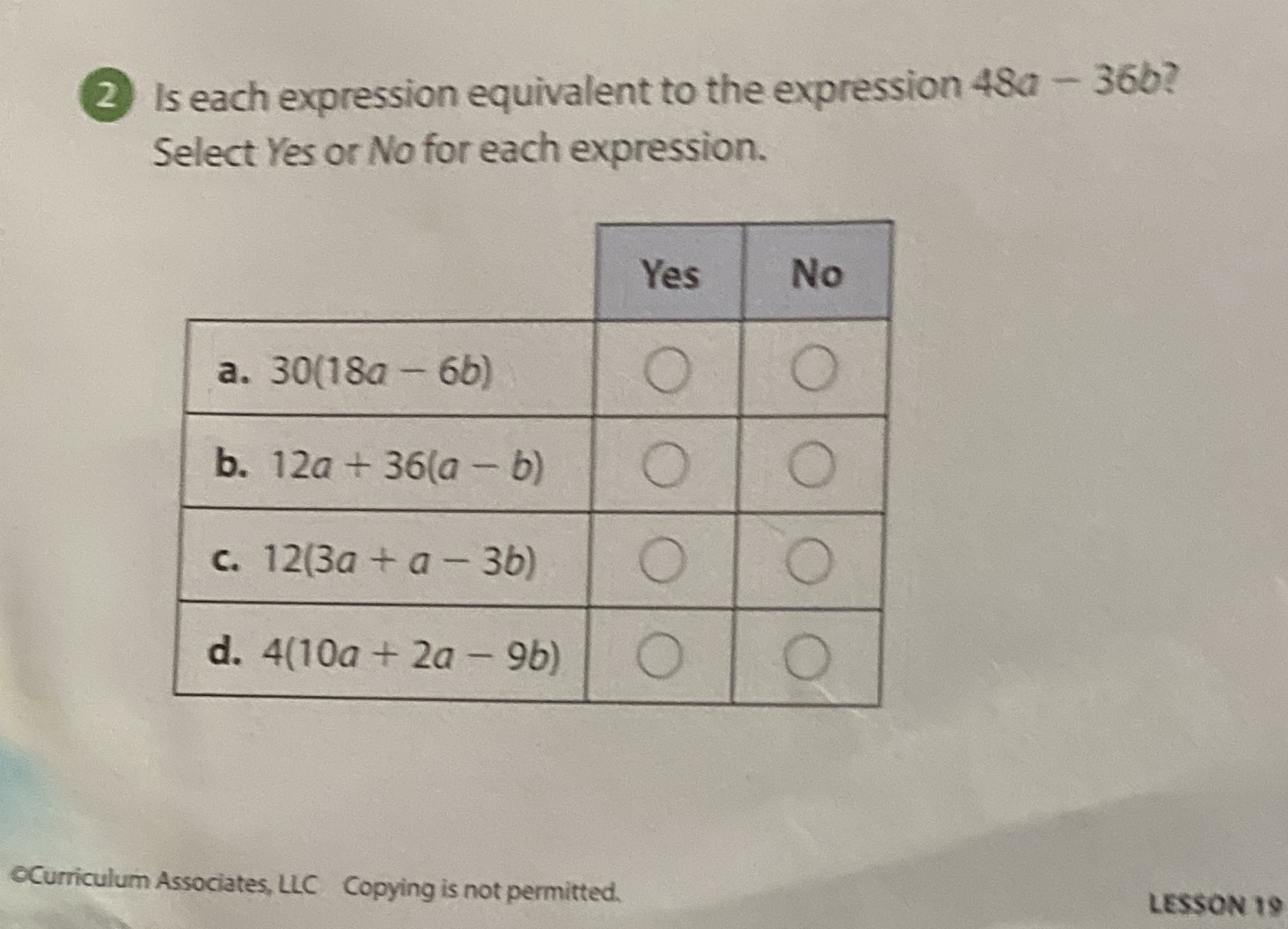### ¿Todavía tienes preguntas de matemáticas?

Pregunte a nuestros tutores expertos
Algebra
Pregunta(2) Is each expression equivalent to the expression $$48 a - 36 b$$ ? Select Yes or No for each expression.

a. $$30 ( 18 a - 6 b )$$

b. $$12 a + 36 ( a - b )$$

c. $$12 ( 3 a + a - 3 b )$$

d. $$4 ( 10 a + 2 a - 9 b )$$